# Image Segmentation on COCO dataset - summary, questions and suggestions

Hi everyone,
I am building an application to segment images around people by using COCO dataset and COCO API.
I tried to follow camvid example but you get lost quite soonAt the moment I can only run the model by keeping one class (which is non sense) and I’m not able to run the model any further to see smart results: probably because I am missing something while building the dataset that gets out later…

I’ll guide you to the problem…
After parsing a while COCO data, I finally had a mask for each file.
By using coco.annToMask I can get mask data and plot it: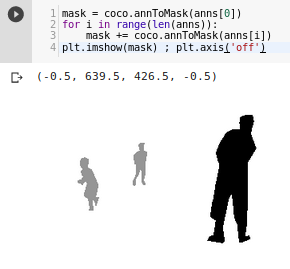Then I create this function to create images for masks (COCO has masks has annotation in RLE), followed by get_y_fn used later to match each file with its mask: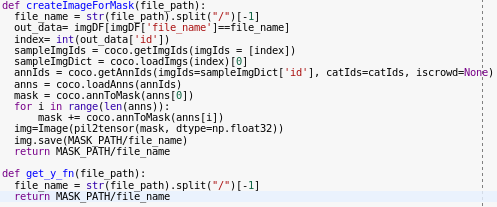If I use the function I get this: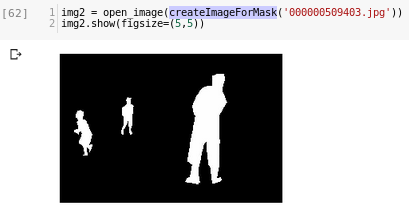B/W image corresponding to the mask.
First question: is this (an image with 2 colours for a 2-class problem) correct?
I think that original data may be in greyscale because children and adults are mapped in different classes…

Then I try to use fastai open_mask method to see results (this will actually be called from show_batch I guess, in fact those images are blue-ish):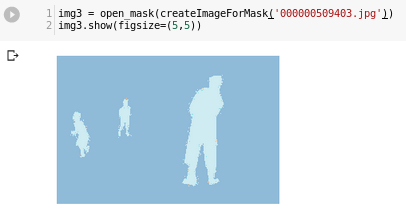Going on… after building databunch by using COCO API, and running show_batch I can see that data is loaded correctly: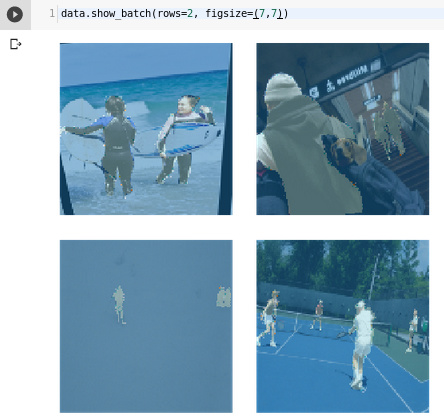Seems right, right?So I continued… At first I only kept 1 category “person” and reached the end of learning process, thanks to these lines:

``````acc_03 = partial(accuracy_thresh, thresh=0.3)
f_score = partial(fbeta, thresh=0.2, beta = 1)

metrics=[acc_03, f_score]
wd=1e-2
learn = unet_learner(data, models.resnet34, metrics=metrics, wd=wd)
learn.loss_func = MSELossFlat()
``````

Second questions: are these metrics and loss appropriate for this application? Suggestions are appreciated.

By showing results I realized that all images had 1 value predicted (the only class that exists).

Going back I added to “classes” a new category “background” and checked how classes and dataset were defined: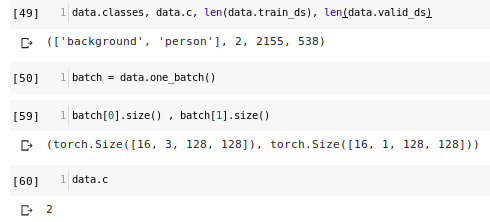As you can see databunch contains tensors with different number of channels (3 vs 1).
Third question: is this ok? (Since mask is greyscale and original data is RGB. But maybe I missed something)

Other image that shows this difference:

If I try to run the model again I get this error:

The size of tensor a (524288) must match the size of tensor b (262144) at non-singleton dimension 0

On forums and blogs people think is caused by the different number of channels, but I don’t understand why this should be the cause, since the code runs if I keep one class (that has the same number of channels as If I put more classes).

Last question: how are classes and pixel values on mask images joined together?
I think that every colour in grey scale has an index (black: 0, … , white: N) and that is joined to the classes array by index: so first class would be black… is this assumption right? (No clue elsewhere…)

So, what should I do? Change the way I create mask image files? add (how) channels to mask images? have a drink?I find it a bit annoying that there is no official tutorial that goes a step beyond the well studied lesson. But this makes things more interesting right?
Anyone happy to help?

6 Likes

Hi everyone,
I fixed the errors and everything works now.
I’ll try to explain the process from the beginning to help new guys face this kind of problem.

My task is binary instance segmentation: say for each pixel wheter it is part of the defined class or not.
In my case class is “person” and dataset comes from COCO. At the moment I’m know interested in evaluate the model with respect to research, so I will simply download the validation data, which is the smaller (containing about 5K images), and use it both for training and validate my first model.

So first of all, download all the data and its annotations.
Then import COCO API :

``````from pycocotools import coco, cocoeval, _mask
from pycocotools import mask as maskUtils
``````

set the category (read COCO metadata if you don’t know them) you are interested in (remember, bynary segmentation -> choose only one):
`CATEGORY_NAMES=['person']`
or
`CATEGORY_NAMES=['dog']`

read COCO annatation file (JSON) and save the images for your category into a dictionary:

``````coco = coco.COCO(ANNOTATION_FILE)
classes = array(TARGET_CLASSES, dtype='<U17')
catIds = coco.getCatIds(catNms=CATEGORY_NAMES);
imgIds = coco.getImgIds(catIds=catIds);
imgDict = coco.loadImgs(imgIds)
len(imgIds) , len(catIds)
``````

I also created a DataFrame to easily access some metadata (there may be an easier way):
`imgDF = pd.DataFrame.from_dict(imgDict)`

I created this function to save the mask of each input file into a new file in MASK_PATH

``````def createImageForMask(file_path):
file_name = str(file_path).split("/")[-1]
out_data= imgDF[imgDF['file_name']==file_name]
index= int(out_data['id'])
sampleImgIds = coco.getImgIds(imgIds = [index])
sampleImgDict = coco.loadImgs(index)
annIds = coco.getAnnIds(imgIds=sampleImgDict['id'], catIds=catIds, iscrowd=None)
anns = coco.loadAnns(annIds)
mask = coco.annToMask(anns)
for i in range(len(anns)):
mask = mask | coco.annToMask(anns[i])
img=Image(pil2tensor(mask, dtype=np.float32))
img.save(MASK_PATH/file_name)
return MASK_PATH/file_name
``````

The key here is using “|” (OR) to collect all pixel values to build the mask. Before this method I found other guys who used “+” (sum) but this would lead to many wrong values in many cells if annotations overlap. At the end of the process we would like a mask that says 0 or 1 for each pixel, i.e. a binary segmentation mask. I read that 0/1 is more efficient than 0/255, maybe someone else could confirm this…

To check if your data is correct you can use this piece of code (pick an ID which is meaningful for you, i.e. you need an image with that ID in your data dir):

``````ID=839
sampleImgIds = coco.getImgIds(imgIds = [ID])
sampleImgDict = coco.loadImgs(sampleImgIds[np.random.randint(0,len(sampleImgIds))])
I = io.imread(sampleImgDict['coco_url'])
plt.imshow(I); plt.axis('off')
annIds = coco.getAnnIds(imgIds=sampleImgDict['id'], catIds=catIds, iscrowd=0)
anns = coco.loadAnns(annIds)
coco.showAnns(anns)
``````

its output:then use this code to check mask creation and pixel values:

``````mask = coco.annToMask(anns)
for i in range(len(anns)):
mask = mask | coco.annToMask(anns[i])
plt.imshow(mask) ; plt.axis('off')

pixVals = set()
for pixRow in mask:
for pix in pixRow:
pixVals.add(pix)
print(pixVals)
``````

its output:as you can see, that 0/1 on top left corner means that all pixels have 0 or 1 as value, that’s fine.

I created this function that can remove from IMG_PATH all files which are not listed in annotation file (to avoid error in databunch) for existing annotations it creates the imageMask:

``````def dataPreparation():
deleted = 0
processed = 0
imgCounter = 0
for f in listdir(IMG_PATH):
imgCounter = imgCounter + 1
if(imgCounter == IMG_COUNT_LIMIT):
break
df = imgDF[imgDF['file_name']==f]
if(df.empty):
#print("delete file: "+f)
os.remove(IMG_PATH/f)
deleted = deleted + 1
else:
createImageForMask(f)
processed = processed + 1
print('deleted '+str(deleted)+' files')
print('processed '+str(processed)+' files')
``````

Now your data should be OK.

create these two utils:

``````class MySegmentationLabelList(SegmentationLabelList):
def open(self, fn): return open_mask(fn, div=True)

class MySegmentationItemList(ImageItemList):
"`ItemList` suitable for segmentation tasks."
_label_cls,_square_show_res = MySegmentationLabelList,False
``````

and define databunch as follows:

``````src = (MySegmentationItemList.from_folder(IMG_PATH)
.random_split_by_pct(.2)
.label_from_func(get_y_fn , classes=TARGET_CLASSES))
tfms = get_transforms()
np.random.seed(23)
data = (src.transform(tfms, size=SZ, tfm_y=True)
.databunch(bs=BS, num_workers=NUM_WORKERS)
.normalize(imagenet_stats))
``````

call show_batch for a first test:

`data.show_batch(rows=2, figsize=(7,7))`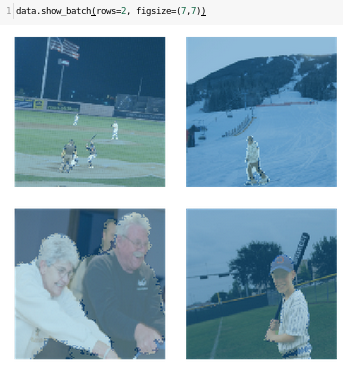Then create model from data bunch:

``````acc_05 = partial(accuracy_thresh, thresh=0.5)
wd=1e-2
learn = unet_learner(data, models.resnet34, wd=wd)
learn.opt_fn=optim.Adam
learn.metrics=[acc_05]
``````

Here I am simply using fastai default loss_function, which should be automatically picked by looking at your dataset class (we are extending ImageItemList with MySegmentationItemList).

From this point you can use all these methods on your model for fine-tuning:

• learn.fit_one_cycle()
• learn.lr_find()
• learn.recorder.plot()

To show your results simply call method show_results:

`learn.show_results(rows=8, figsize=(25, 25))`

here a simple output (left is actual value, right is predicted):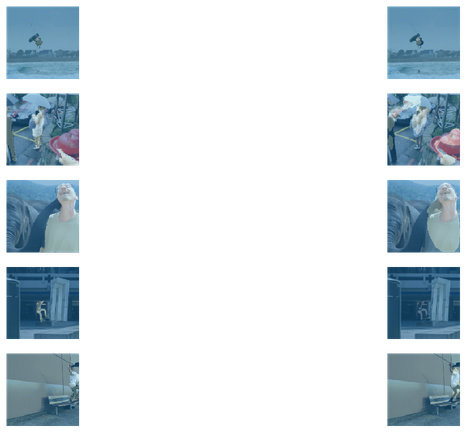As you can see there is a segmentation mask on some people on the right, so it’s workingYou can now work with coco dataset for binary segmentation.

Next step can be to identify more than one category, we need:

• a proper way to define masks for many classes

• both loss_function and metrics to handle more than one class

bye7 Likes

have you tried using the metric from camvid? im pretty sure thats the classification accuracy per pixel which would be good for what youre doing (and i think its different than the metric youre using).

also, (not sure if this is what you have) the tensors of the labels/masks images you have should be the class of each pixel. so they should probably be all 0’s and 1’s since youre just predicting masks for humans.

i did this for the camvid dataset to turn it into the dataset from the tiramisu paper, so i might be able to help if you run into any problems

The issue with image masks being black and white if you try doing multiclass may be due to using PIL L mode when you should instead use P mode. Any chance you’ve figured out how to use the json data directly instead of needing to create the intermediary mask images?

Can anyone think of a way to show the “top losses” for a segmentation problem?

What I’m trying to do is getting the worst performers, manually annotate and include them in the training set.

Besides, is that a good strategy?

Maybe you could add the losses of each pixel and sum these up? Obviously, this won’t be as good a predictor for “worst performance” as in a classification task, but sounds reasonable.

If you try I would be interested in the resultsHow can I predict a single image using fastai after training like this?

1 Like

You could export your model as a pickle file using learn.export(). Later for inference learner = load_learner() ie, load back the pickle file. Read in the single image using open_image(path). Use this to do the prediction using learn.predict().

1 Like

Thank you! I’ll try that later

Do you have a proper way to define masks for many classes?Hi Pietro, I need to same function. but it does not work for me. It gives key error:0
How can I solve it?

@pietro.latorre
Thank you for posting this useful and guidable post, it helps a lot. Can you please collaborate and explain how to handle a multiclass dataset (2,3 classes or more)?